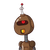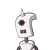# All angles of a 12 sided polygon are the same. How much is each ?​

All angles of a 12 sided polygon are the same. How much is each ?​

### 2 thoughts on “All angles of a 12 sided polygon are the same. How much is each ?​”

1.150

Step-by-step explanation:

Sum of the interior angles = (n-2)180

= (12-2)180

= 1800

n= number of sides

Each angle = 1800/12 = 150

2.